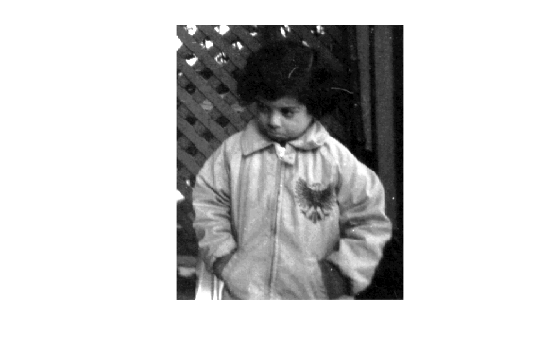# stretchlim

Find limits to contrast stretch image

## Syntax

``lowhigh = stretchlim(I)``
``lowhigh = stretchlim(I,Tol)``

## Description

example

````lowhigh = stretchlim(I)` computes the lower and upper limits that can be used for contrast stretching grayscale or RGB image `I`. The limits are returned in `lowhigh`. By default, the limits specify the bottom 1% and the top 1% of all pixel values.```
````lowhigh = stretchlim(I,Tol)` specifies the fraction, `Tol`, of the image to saturate at low and high pixel values.```

## Examples

collapse all

Read grayscale image into the workspace and display it.

```I = imread('pout.tif'); figure imshow(I)```Adjust the contrast in the image using `stretchlim` to set the limits, and display the result. The example uses the default limits `[0.01 0.99]`, saturating the upper 1% and the lower 1%.

```J = imadjust(I,stretchlim(I),[]); figure imshow(J)```## Input Arguments

collapse all

Image to be contrast stretched, specified as a 2-D grayscale image or 2-D RGB image.

Data Types: `single` | `double` | `int16` | `uint8` | `uint16`

Fraction of the image to saturate, specified as a numeric scalar or 2-element vector `[Low_Fract High_Fract]` in the range [0, 1].

ValueDescription
ScalarIf `Tol` is a scalar, then `Low_Fract = Tol`, and `High_Fract = 1 - Low_Fract`, which saturates equal fractions at low and high pixel values.
0If `Tol = 0`, then ```lowhigh = [min(I(:)); max(I(:))]```.
DefaultIf you omit the `Tol` argument, then `[Low_Fract High_Fract]` defaults to `[0.01 0.99]`, saturating 2%.
Too bigIf `Tol` is too big, such that no pixels would be left after saturating low and high pixel values, then `stretchlim` returns ```[0 1]```.

Example: `[.02 .80]`

Data Types: `single` | `double` | `int8` | `int16` | `int32` | `int64` | `uint8` | `uint16` | `uint32` | `uint64`

## Output Arguments

collapse all

Lower and upper limits for contrast stretching, returned as one of the following.

• A 2-element numeric vector when `I` is a grayscale image.

• A 2-by-3 numeric matrix when `I` is an RGB image. The columns indicate the lower and upper limit for each of the three color channels.

Data Types: `double`

## Version History

Introduced before R2006a